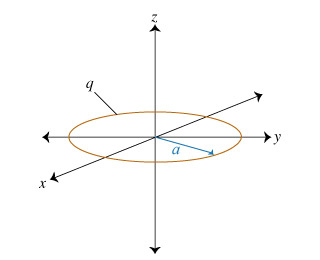# Problem: Consider a uniformly charged ring in the xy plane, centered at the origin. The ring has radius a and positive charge q distributed evenly along its circumference. Imagine a small metal ball of mass m and negative charge -q0. The ball is released from rest at the point (0, 0, d) and constrained to move along the z-axis, with no damping. If 0 &lt; d &lt;&lt; a, what will be the ball's subsequent trajectory?A. repelled from the originB. attracted toward the origin and coming to restC.oscillatingalong the z-axis between z = d and z = -d.D. circling around the z-axis at z =d

###### FREE Expert Solution

Electric force:

$\overline{){\mathbf{F}}{\mathbf{=}}\frac{{\mathbf{kq}}_{\mathbf{1}}{\mathbf{q}}_{\mathbf{2}}}{{\mathbf{r}}^{\mathbf{2}}}}$

Newton's second law:

$\overline{){\mathbf{F}}{\mathbf{=}}{\mathbit{m}}{\mathbit{a}}}$

q = -q0

E = kqz/(z2 + a2)(3/2)

F = -kqq0z/(z2 + a2)(3/2)

at a point z = d,

F = -kqq0d/(d2 + a2)(3/2)

When d << a, (d2 + a2) is approximately equal to a2

F = -kqq0d/a3 = (-kqq0/a3)d

80% (3 ratings)###### Problem Details

Consider a uniformly charged ring in the xy plane, centered at the origin. The ring has radius a and positive charge q distributed evenly along its circumference.Imagine a small metal ball of mass m and negative charge -q0. The ball is released from rest at the point (0, 0, d) and constrained to move along the z-axis, with no damping. If 0 < d << a, what will be the ball's subsequent trajectory?

A. repelled from the origin

B. attracted toward the origin and coming to rest

C.oscillatingalong the z-axis between z = d and z = -d.

D. circling around the z-axis at z =d